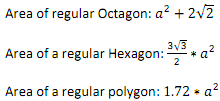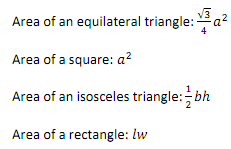### Compute area and perimeter of a polygon

Assignment Help JAVA Programming
##### Reference no: EM131829

The goal is to create a project that would let a user compute area and perimeter of a polygon. Restrict the type of polygon inputs to Triangle, Quadrilateral, Pentagon, Hexagon, and Octagon only.

Your program must implement classes IsocelesTriangle, EquilateralTriangle, Rectangle, and Square, which have the appropriate inheritance relationships. Have functionality that tests if two polygons are similar.

Give the type of polynomial and its coordinates as inputs. Use abstract classes, interfaces, and exception handling where appropriate.

For pentagon, hexagon, and octagon assume that the polygon is regular i.e. all the sides are of same length. For these the input must consist of the type of polygon and length of side (example: pentagon and 7 where type is pentagon and 7 is length of each side of a pentagon). Use the following formulae's to compute the area of a regular polygon where "a" is the length of the side.In case of a triangle or quadrilateral you may or may not have a regular polygon. If a triangle or quadrilateral is regular then it's an equilateral triangle or a square respectively. In these cases again your input will consist of the type of polygon and length of side (example: quadrilateral and 4). If a triangle or quadrilateral is not regular then the only allowed types must be an rectangle and isosceles triangle. In each of these cases your inputs will consist of three parts. The first part will be type of polygon and second and third parts will be the two different possible side. In case of a rectangle let your second input be length (l) and third input be width (w) and in case of a isosceles triangle let the second input be base(b) and the third input be height (h). Use the following formulae's for triangles and quadrilaterals.### Write a Review